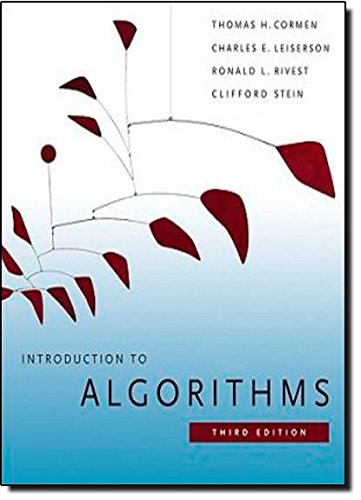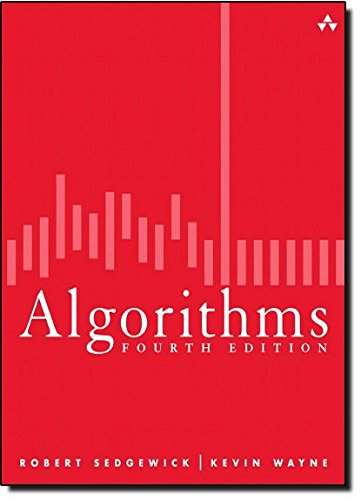# Best Algorithms and Data Structure Book for Enriching Your Analyzing Capability with Practical Approaches

The algorithm is one of the most effective for solving any programming based problem. It not only save your times for solving a problem but also make your program more robust. Also, the data structure helps a program to organize, recognize, storage format and management of data effectively. To become an excellent programmer, it is essential to learn Algorithms and Data Structures. Here are some 7 best algorithms and data structure book to guide you through learning Data Structures and Algorithms.

## 1. The Algorithm Design Manual

This book addresses the main difficulties of solving problems and provides straightforward access to combinatorial algorithm technology, stressing design over analysis. It covers hundreds of practical techniques that give an overview of nearly every type of algorithmic problems we encounter.

What you will learn

• Essentials to understand the algorithmic design
• How to estimate the resources required for an algorithm
• Fundamental and specialized data structure concepts with stack, queues, linked lists, and graphs
• How to choose the best sorting algorithm for different sorting situations
• Searching with classical, graphical, and heuristic methods
• Applications of dynamic programming
• Step by step procedures for solving common algorithmic problems with 75 solved examples

## 2. Introduction to Algorithms, 3rd Edition (MIT Press)Author: Thomas H. Cormen,Charles E. Leiserson,Ronald L. Rivest,Clifford Stein
Published at: 31/07/2009
ISBN: 0262033844

This book covers a broad range of algorithms in depth with simple and accessible design methods. That makes it a useful book for all the levels of the programmer from graduate student to working professional. It explains the concepts using the pseudo code but that can be transferred to any programming language without much struggle.

What you will learn

• Methods for solving recurrences
• Probabilistic analysis and further uses of indicator random variables
• Advanced data structures using binary search, Fibonacci heaps, Van Emden Boas trees
• Sorting and ordered statistics
• Elementary and advanced graph algorithms
• String matching and multithreaded algorithms
• Computational geometry and approximation algorithms
e-height: 26px;">This book covers a broad range of algorithms in depth with simple and accessible design methods. That makes it a useful book for all the levels of the programmer from graduate student to working professional. It explains the concepts using the pseudo code but that can be transferred to any programming language without much struggle.

What you will learn

• Methods for solving recurrences
• Probabilistic analysis and further uses of indicator random variables
• Advanced data structures using binary search, Fibonacci heaps, Van Emden Boas trees
• Sorting and ordered statistics
• Elementary and advanced graph algorithms
• String matching and multithreaded algorithms
• Computational geometry and approximation algorithms

## 3. Algorithms (4th Edition)Author: Robert Sedgewick,Kevin Wayne
Published at: 19/03/2011
ISBN: 032157351X

Algorithms (4th Edition) is the leading textbook and is widely used worldwide by graduate and undergraduate students. This book surveys the most important computer algorithms currently in use and provides in-depth analysis of most useful algorithms and data structures. The codes are written in Java where all of the codes are exposed to the reader and ready to use.

What you will learn

• Basic programming model and data abstraction
• Applications of different sorting algorithms
• Searching-using symbol tables, hash tables, and binary search tree
• String sorts and substring search
• Applications of graph algorithms for shortest paths and minimum spanning trees
• Event-driven simulation using hand disc model
• Network flow and reduction

## 4. Data Structures and Algorithms Made Easy: Data Structures and Algorithmic Puzzles, Fifth Edition

This book is an effective solution for complex data structures and algorithms. It contains various data structures and algorithm-related complex problems with appropriate solutions that are useful for readers studying computer science.

What you will learn

• Fundamentals of Data Structures and Algorithms
• Applications of linked lists over arrays and dynamic arrays
• How to solve a problem with recursion and without recursion(using stacks)
• Selection algorithms for finding medians
• Priority queues and heaps
• Divide and conquer algorithms for efficient sorting and searching
• Hashing and string algorithms for working with strings

## 5. Algorithms Unlocked (MIT Press)

This book offers an engagingly written guide to the basics of computer algorithms. It provides a general explanation, with limited mathematics, of how algorithms enable computers to solve problems. Readers will learn what computer algorithms are, how to describe them, and how to evaluate them.

What you will learn

• Simple ways to search information in a computer
• Methods for rearranging data in a prescribed order
• How to solve problems that ask questions about strings of textual characters
• The basic principles behind cryptography
• Fundamentals of data compression
• Simple solutions to seemingly hard problems

## 6. The Art of Computer Programming, Vol. 1: Fundamental Algorithms, 3rd Edition

The Art of Computer Programming provides a comprehensive overview of a wide variety of algorithms and the analysis with clarity, accuracy, and good humor. Scientists have marveled at the beauty and elegance of this book while practicing programmers have successfully applied this solution to their day-to-day problems.

What you will learn

• Generating basic combinatorial patterns in permutation and combination
• Clause learning algorithms
• Monte Carlo algorithms
• Satisfiability-preserving maps
• Dancing links for backtrack programming
• Message passing algorithms

This book presents a comprehensive look at the ideas, analysis, and implementation details of data structures as a specialized topic in applied algorithms. It contains numerous code examples in C and more than 500 references that makes it an essential book for the learners of computer programing.

What you will learn

• Elementary data structures with stacks and queues
• Basic search trees and balanced search trees
• Tree structures for sets of intervals
• Different applications of heap structures
• How to transform data structures
• Data structures for strings
• Basic hash tables and perfect hash functions

## 8. Grokking Algorithms: An illustrated guide for programmers and other curious people

This amazing book gives you complete details and shows you how to apply common algorithms in practical problems which you face every day. It can help you to sort, search and think algorithmically. Also, you can tackle more complex concerns such as data compression and artificial intelligence. It includes many helpful diagrams and fully annotated code as a sample in python.

What you'll learn-

• Search, sort and graph algorithms
• 400 pictures with detailed work
• Python-based code samples
• Selection, recursion and quicksort
• Hash tables and breadth-first search
• Dijkstra's and greedy  algorithm
• Dynamic programming
• K-nearest neighbors

## 9. A Common-Sense Guide to Data Structures and Algorithms: Level Up Your Core Programming Skills

This book gives you many interesting topics on algorithms and data structures. It enables you to write code that runs faster and more efficiently. It has a practical approach to data structures and algorithms, with techniques and real-world scenarios that you can use in your daily production code. Graphics and examples consist of this book can easily understandable to you.

What you'll learn-

• Evaluate algorithms and articulation of your code
• Ways to solve a tricky problem
• How to choice arrays and linked list
• Hash tables
• Binary trees and graphs